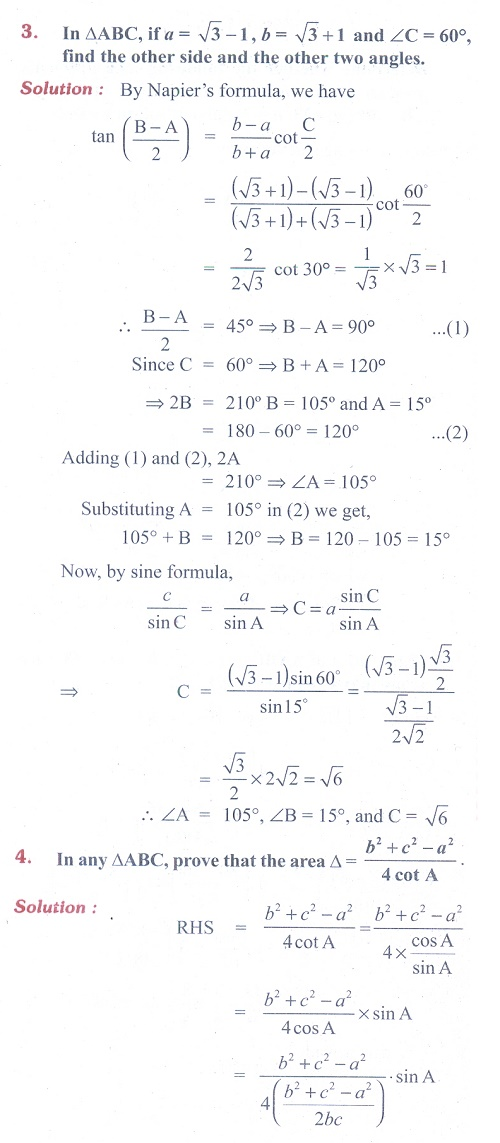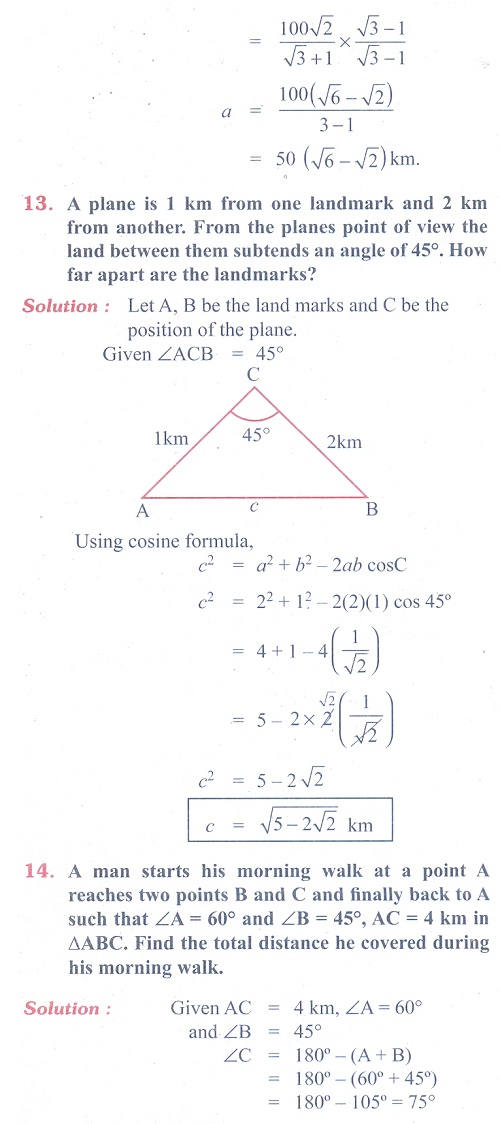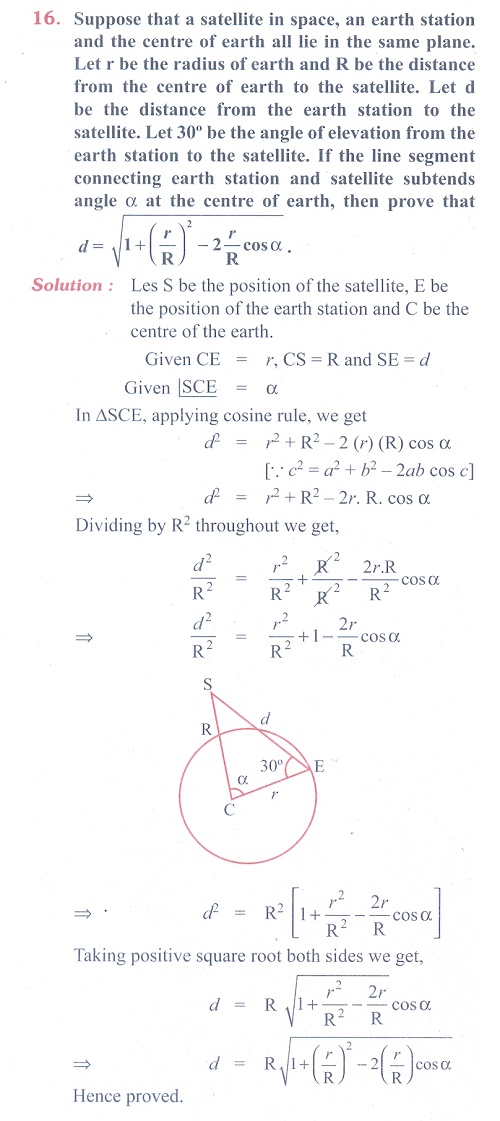Home | | Maths 11th std | Exercise 3.10: Trigonometry Application to Triangle

# Exercise 3.10: Trigonometry Application to Triangle

Maths Book back answers and solution for Exercise questions - Mathematics : Trigonometry: Application to TriangleTags : Problem Questions with Answer, Solution | Trigonometry | Mathematics , 11th Mathematics : UNIT 3 : Trigonometry
Study Material, Lecturing Notes, Assignment, Reference, Wiki description explanation, brief detail
11th Mathematics : UNIT 3 : Trigonometry : Exercise 3.10: Trigonometry Application to Triangle | Problem Questions with Answer, Solution | Trigonometry | Mathematics